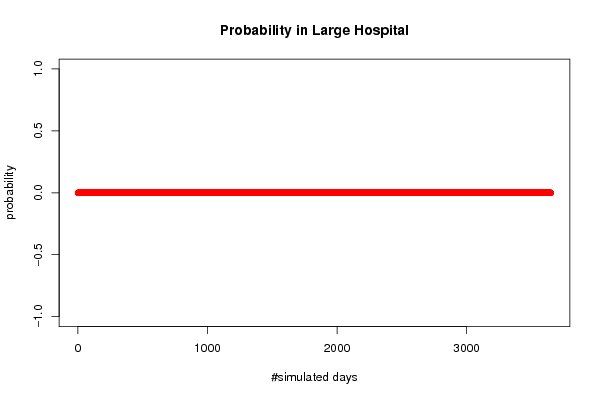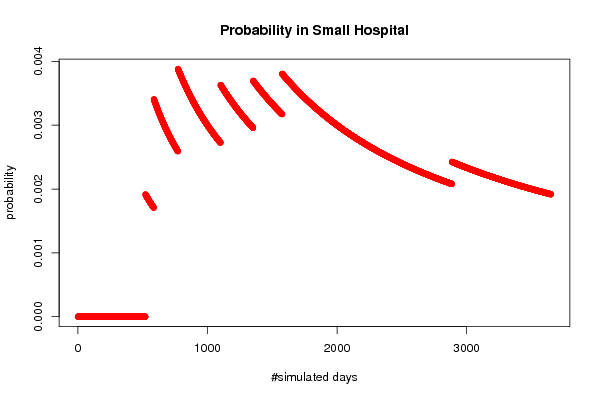## Free Statistics

of Irreproducible Research!

Author's title
Author*Unverified author*
R Software Modulerwasp_babies.wasp
Title produced by softwareExercise 1.13
Date of computationFri, 09 Oct 2009 07:06:48 -0600
Cite this page as followsStatistical Computations at FreeStatistics.org, Office for Research Development and Education, URL https://freestatistics.org/blog/index.php?v=date/2009/Oct/09/t1255093679ens4mnoygdppn14.htm/, Retrieved Thu, 06 Oct 2022 01:47:42 +0000
Statistical Computations at FreeStatistics.org, Office for Research Development and Education, URL https://freestatistics.org/blog/index.php?pk=45058, Retrieved Thu, 06 Oct 2022 01:47:42 +0000
QR Codes:Original text written by user:
IsPrivate?No (this computation is public)
User-defined keywords
Estimated Impact107
Family? (F = Feedback message, R = changed R code, M = changed R Module, P = changed Parameters, D = changed Data)
-     [Exercise 1.13] [vraag 1 ws 1(2)] [2009-10-09 12:30:05] [66ab305790aa3940f54dc38969d76367]
-   P   [Exercise 1.13] [ws 1 vraag 2] [2009-10-09 12:51:28] [66ab305790aa3940f54dc38969d76367]
-   P       [Exercise 1.13] [vraag 3 ws 1] [2009-10-09 13:06:48] [cbe9d377b32b54bb6800c19daa3246d2] [Current]
-             [Exercise 1.13] [vraag 3 ws 2] [2009-10-09 13:12:40] [66ab305790aa3940f54dc38969d76367]
Feedback Forum

Post a new message

 Summary of computational transaction Raw Input view raw input (R code) Raw Output view raw output of R engine Computing time 7 seconds R Server 'Sir Ronald Aylmer Fisher' @ 193.190.124.24

\begin{tabular}{lllllllll}
\hline
Summary of computational transaction \tabularnewline
Raw Input & view raw input (R code)  \tabularnewline
Raw Output & view raw output of R engine  \tabularnewline
Computing time & 7 seconds \tabularnewline
R Server & 'Sir Ronald Aylmer Fisher' @ 193.190.124.24 \tabularnewline
\hline
\end{tabular}
%Source: https://freestatistics.org/blog/index.php?pk=45058&T=0

[TABLE]
[ROW][C]Summary of computational transaction[/C][/ROW]
[ROW][C]Raw Input[/C][C]view raw input (R code) [/C][/ROW]
[ROW][C]Raw Output[/C][C]view raw output of R engine [/C][/ROW]
[ROW][C]Computing time[/C][C]7 seconds[/C][/ROW]
[ROW][C]R Server[/C][C]'Sir Ronald Aylmer Fisher' @ 193.190.124.24[/C][/ROW]
[/TABLE]
Source: https://freestatistics.org/blog/index.php?pk=45058&T=0

Globally Unique Identifier (entire table): ba.freestatistics.org/blog/index.php?pk=45058&T=0

As an alternative you can also use a QR Code:

The GUIDs for individual cells are displayed in the table below:

 Summary of computational transaction Raw Input view raw input (R code) Raw Output view raw output of R engine Computing time 7 seconds R Server 'Sir Ronald Aylmer Fisher' @ 193.190.124.24

 Exercise 1.13 p. 14 (Introduction to Probability, 2nd ed.) Number of simulated days 3650 Expected number of births in Large Hospital 45 Expected number of births in Small Hospital 15 Percentage of Male births per day(for which the probability is computed) 0.8 #Females births in Large Hospital 82065 #Males births in Large Hospital 82185 #Female births in Small Hospital 27464 #Male births in Small Hospital 27286 Probability of more than 80 % of male births in Large Hospital 0 Probability of more than 80 % of male births in Small Hospital 0.00191780821917808 #Days per Year when more than 80 % of male births occur in Large Hospital 0 #Days per Year when more than 80 % of male births occur in Small Hospital 0.7

\begin{tabular}{lllllllll}
\hline
Exercise 1.13 p. 14 (Introduction to Probability, 2nd ed.) \tabularnewline
Number of simulated days & 3650 \tabularnewline
Expected number of births in Large Hospital & 45 \tabularnewline
Expected number of births in Small Hospital & 15 \tabularnewline
Percentage of Male births per day(for which the probability is computed) & 0.8 \tabularnewline
#Females births in Large Hospital & 82065 \tabularnewline
#Males births in Large Hospital & 82185 \tabularnewline
#Female births in Small Hospital & 27464 \tabularnewline
#Male births in Small Hospital & 27286 \tabularnewline
Probability of more than 80 % of male births in Large Hospital & 0 \tabularnewline
Probability of more than 80 % of male births in Small Hospital & 0.00191780821917808 \tabularnewline
#Days per Year when more than 80 % of male births occur in Large Hospital & 0 \tabularnewline
#Days per Year when more than 80 % of male births occur in Small Hospital & 0.7 \tabularnewline
\hline
\end{tabular}
%Source: https://freestatistics.org/blog/index.php?pk=45058&T=1

[TABLE]
[ROW][C]Exercise 1.13 p. 14 (Introduction to Probability, 2nd ed.)[/C][/ROW]
[ROW][C]Number of simulated days[/C][C]3650[/C][/ROW]
[ROW][C]Expected number of births in Large Hospital[/C][C]45[/C][/ROW]
[ROW][C]Expected number of births in Small Hospital[/C][C]15[/C][/ROW]
[ROW][C]Percentage of Male births per day(for which the probability is computed)[/C][C]0.8[/C][/ROW]
[ROW][C]#Females births in Large Hospital[/C][C]82065[/C][/ROW]
[ROW][C]#Males births in Large Hospital[/C][C]82185[/C][/ROW]
[ROW][C]#Female births in Small Hospital[/C][C]27464[/C][/ROW]
[ROW][C]#Male births in Small Hospital[/C][C]27286[/C][/ROW]
[ROW][C]Probability of more than 80 % of male births in Large Hospital[/C][C]0[/C][/ROW]
[C]Probability of more than 80 % of male births in Small Hospital[/C][C]0.00191780821917808[/C][/ROW]
[ROW][C]#Days per Year when more than 80 % of male births occur in Large Hospital[/C][C]0[/C][/ROW]
[C]#Days per Year when more than 80 % of male births occur in Small Hospital[/C][C]0.7[/C][/ROW]
[/TABLE]
Source: https://freestatistics.org/blog/index.php?pk=45058&T=1

Globally Unique Identifier (entire table): ba.freestatistics.org/blog/index.php?pk=45058&T=1

As an alternative you can also use a QR Code:

The GUIDs for individual cells are displayed in the table below:

 Exercise 1.13 p. 14 (Introduction to Probability, 2nd ed.) Number of simulated days 3650 Expected number of births in Large Hospital 45 Expected number of births in Small Hospital 15 Percentage of Male births per day(for which the probability is computed) 0.8 #Females births in Large Hospital 82065 #Males births in Large Hospital 82185 #Female births in Small Hospital 27464 #Male births in Small Hospital 27286 Probability of more than 80 % of male births in Large Hospital 0 Probability of more than 80 % of male births in Small Hospital 0.00191780821917808 #Days per Year when more than 80 % of male births occur in Large Hospital 0 #Days per Year when more than 80 % of male births occur in Small Hospital 0.7PNG link Postscript link PDF linkPNG link Postscript link PDF link

Parameters (Session):
par1 = 3650 ; par2 = 45 ; par3 = 15 ; par4 = 0.8 ;
Parameters (R input):
par1 = 3650 ; par2 = 45 ; par3 = 15 ; par4 = 0.8 ;
R code (references can be found in the software module):
par1 <- as.numeric(par1)par2 <- as.numeric(par2)par3 <- as.numeric(par3)par4 <- as.numeric(par4)numsuccessbig <- 0numsuccesssmall <- 0bighospital <- array(NA,dim=c(par1,par2))smallhospital <- array(NA,dim=c(par1,par3))bigprob <- array(NA,dim=par1)smallprob <- array(NA,dim=par1)for (i in 1:par1) {bighospital[i,] <- sample(c('F','M'),par2,replace=TRUE)if (as.matrix(table(bighospital[i,])) > par4*par2) numsuccessbig = numsuccessbig + 1bigprob[i] <- numsuccessbig/ismallhospital[i,] <- sample(c('F','M'),par3,replace=TRUE)if (as.matrix(table(smallhospital[i,])) > par4*par3) numsuccesssmall = numsuccesssmall + 1smallprob[i] <- numsuccesssmall/i}tbig <- as.matrix(table(bighospital))tsmall <- as.matrix(table(smallhospital))tbigtsmallnumsuccessbig/par1bigprob[par1]numsuccesssmall/par1smallprob[par1]numsuccessbig/par1*365bigprob[par1]*365numsuccesssmall/par1*365smallprob[par1]*365bitmap(file='test1.png')plot(bigprob,col=2,main='Probability in Large Hospital',xlab='#simulated days',ylab='probability')dev.off()bitmap(file='test2.png')plot(smallprob,col=2,main='Probability in Small Hospital',xlab='#simulated days',ylab='probability')dev.off()load(file='createtable')a<-table.start()a<-table.row.start(a)a<-table.element(a,'Exercise 1.13 p. 14 (Introduction to Probability, 2nd ed.)',2,TRUE)a<-table.row.end(a)a<-table.row.start(a)a<-table.element(a,'Number of simulated days',header=TRUE)a<-table.element(a,par1)a<-table.row.end(a)a<-table.row.start(a)a<-table.element(a,'Expected number of births in Large Hospital',header=TRUE)a<-table.element(a,par2)a<-table.row.end(a)a<-table.row.start(a)a<-table.element(a,'Expected number of births in Small Hospital',header=TRUE)a<-table.element(a,par3)a<-table.row.end(a)a<-table.row.start(a)a<-table.element(a,'Percentage of Male births per day(for which the probability is computed)',header=TRUE)a<-table.element(a,par4)a<-table.row.end(a)a<-table.row.start(a)a<-table.element(a,'#Females births in Large Hospital',header=TRUE)a<-table.element(a,tbig)a<-table.row.end(a)a<-table.row.start(a)a<-table.element(a,'#Males births in Large Hospital',header=TRUE)a<-table.element(a,tbig)a<-table.row.end(a)a<-table.row.start(a)a<-table.element(a,'#Female births in Small Hospital',header=TRUE)a<-table.element(a,tsmall)a<-table.row.end(a)a<-table.row.start(a)a<-table.element(a,'#Male births in Small Hospital',header=TRUE)a<-table.element(a,tsmall)a<-table.row.end(a)a<-table.row.start(a)dum1 <- paste('Probability of more than', par4*100, sep=' ')dum <- paste(dum1, '% of male births in Large Hospital', sep=' ')a<-table.element(a, dum, header=TRUE)a<-table.element(a, bigprob[par1])a<-table.row.end(a)dum <- paste(dum1, '% of male births in Small Hospital', sep=' ')a<-table.element(a, dum, header=TRUE)a<-table.element(a, smallprob[par1])a<-table.row.end(a)a<-table.row.start(a)dum1 <- paste('#Days per Year when more than', par4*100, sep=' ')dum <- paste(dum1, '% of male births occur in Large Hospital', sep=' ')a<-table.element(a, dum, header=TRUE)a<-table.element(a, bigprob[par1]*365)a<-table.row.end(a)dum <- paste(dum1, '% of male births occur in Small Hospital', sep=' ')a<-table.element(a, dum, header=TRUE)a<-table.element(a, smallprob[par1]*365)a<-table.row.end(a)a<-table.end(a)table.save(a,file='mytable.tab')